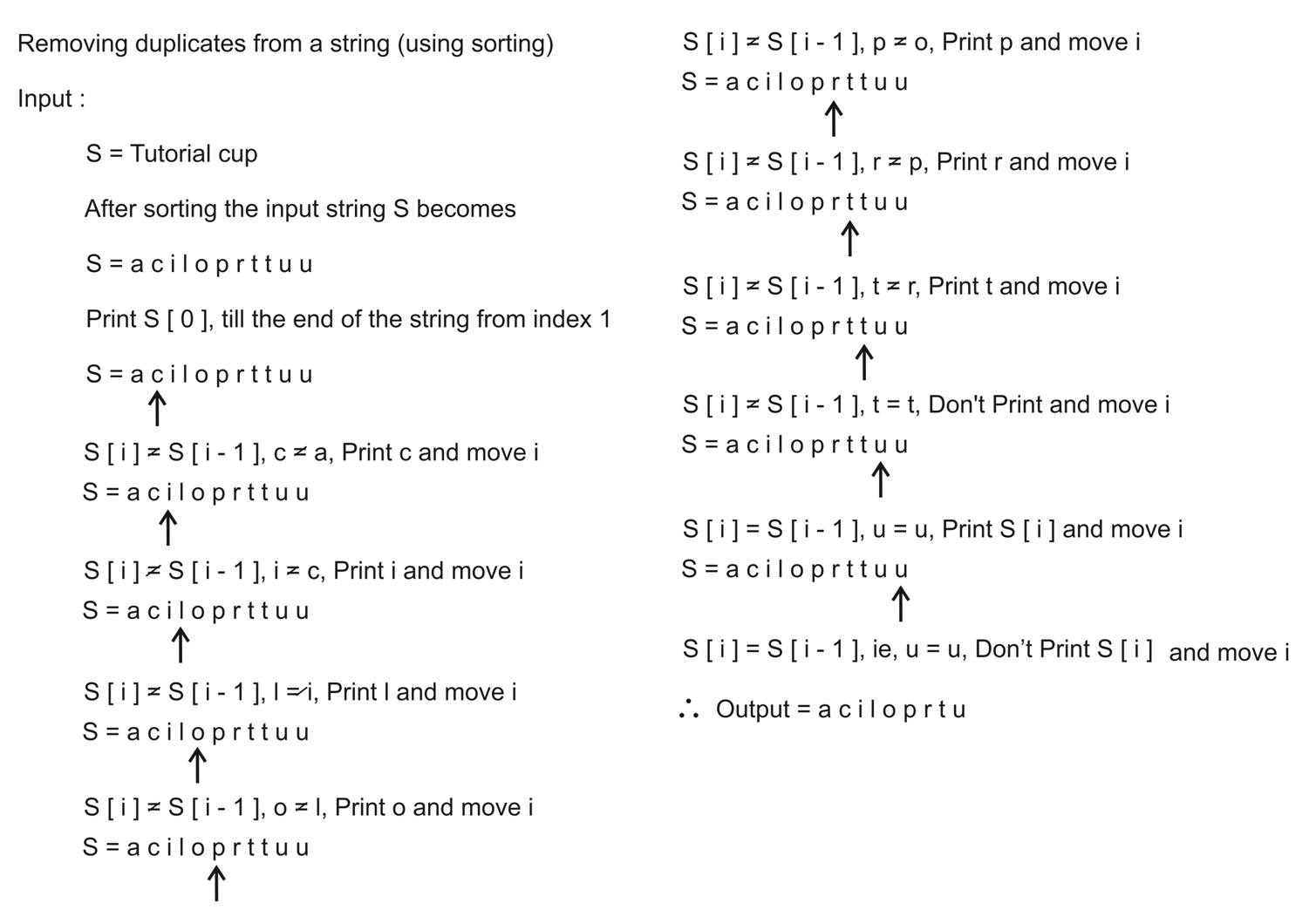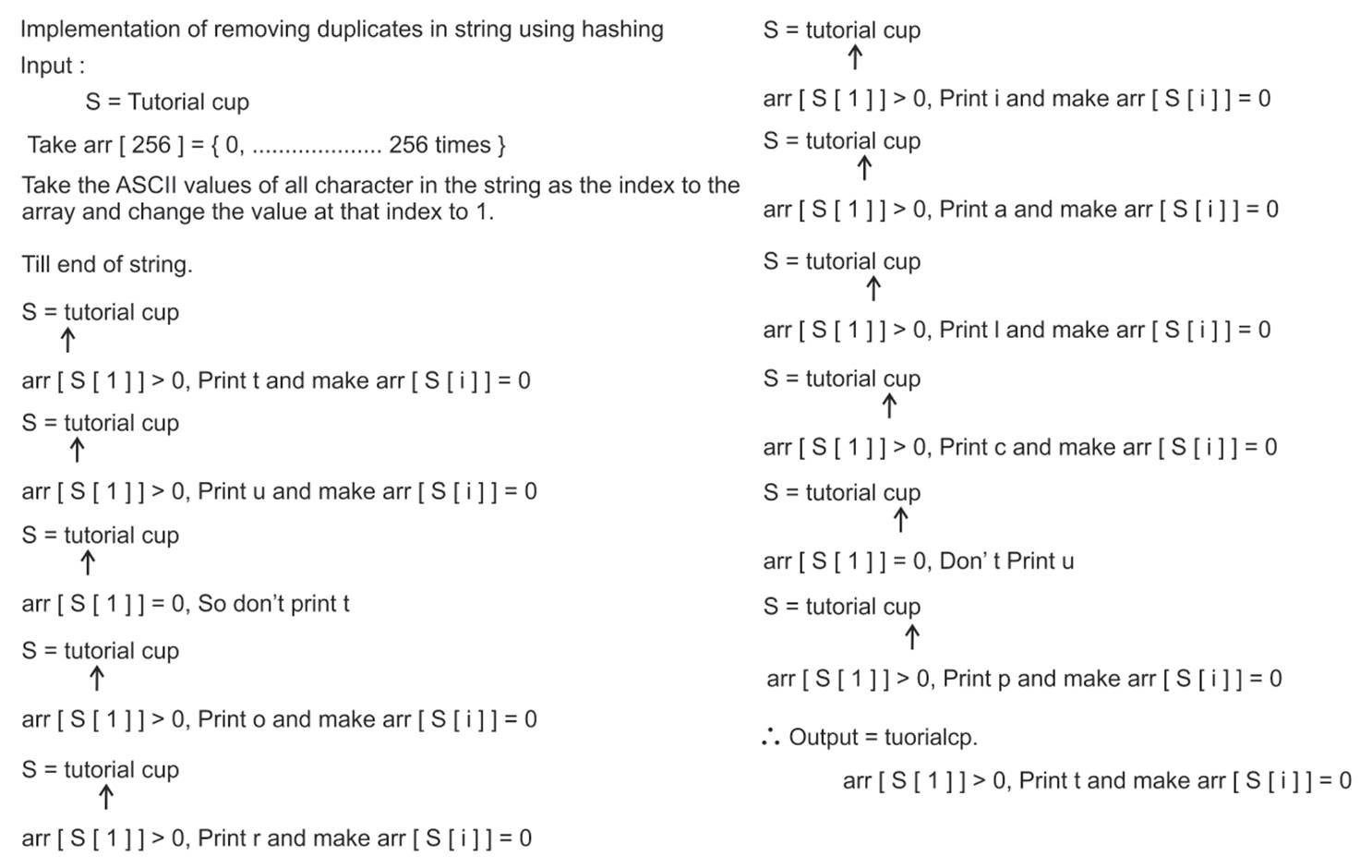# Remove duplicates from a string

StringViews 3854

## Given a string, this function removes all the duplicates in the string and prints the string which has no duplcates. Here, duplicates are the elements which occur more than once in the string

Time Complexity: O(nlogn),

if we use some nlogn sorting algorithm

## Algorithm1(Using Sorting)

### Example

INPUT

s = tutorialcup

OUTPUT

aciloprtu

In the above example, when we use the sorting algorithm then aciloprtu is the output string

In this method we will sort the input string and will compare the adjacent elements.
1. Sort the input string ie, use sort function sort(s.begin(), s.end())
2.  Print the first character
3. From the first index till the end of the string, compare the currennt character to the previous character. If it is same, then the character is duplicate

## C++ Program

```#include <bits/stdc++.h>
using namespace std;

int main()
{
string s; //two string
cin>>s;

sort(s.begin(),s.end()); //Sort the string

cout<<s; //print the first element

for(int i=1; i<s.size(); i++) // start from the first index till end and check if the character has occured once before
if(s[i] != s[i-1])
cout <<s[i];

return 0;
}```Time Complexity : O(n)

## Algorithm 2(using hashing)

### Example

INPUT
s = tutorialcup

OUTPUT
tuorialcp

In the above example, when we use the hashing algorithm then tuorialcp is the output string

In this method we will use hashing and remove all the duplicates in the input string
1. Create an array of size 256 and intialize it to zero. ie, 256 ASCII characters, count=0 for each character
2. Increment the count of each character by using ASCII of character as key ie, arr[s[i]]++ here, s[i] gives the ASCII of the present character
3. Till end of the string, check the count of the each character
a. If the count of the character is greater than zero, then print the character and set the count equal to zero

## C++ Program

```#include <bits/stdc++.h>
using namespace std;
#define NO_OF_CHARS 256
int main()
{
string s; //two string
cin>>s;

int arr[NO_OF_CHARS]; // hash the characters
memset(arr,0,sizeof(arr)); //initialize the count of each character to zero

for(int i=0; i<s.size(); i++)
arr[s[i]]++; //increment the count of each character by using ASCII of character as key
for(int i=0; i<s.size(); i++)
if(arr[s[i]] > 0) //if character has occured then print it once and set its count to zero so that we dont print it again while traversing the array
{
cout<<s[i];
arr[s[i]] = 0;
}

return 0;
}```Translate »